Skip to content
Related Articles
Pandas filter a dataframe by the sum of rows or columns
• Last Updated : 16 Mar, 2021

In this article, we will see how to filter a Pandas DataFrame by the sum of rows or columns. This can be useful in some conditions. Let’s suppose you have a data frame consisting of customers and their purchased fruits.  The rows consist of different customers and columns contain different types of fruits. You want to filter the data frame on the basis of their purchasing. To know more about filter Pandas DataFrame by column values and rows based on conditions refer to the article links. Pandas dataframe.sum() function has been used to return the sum of the values.

Steps needed:

1. Create or import the data frame
2. Sum the rows: This can be done using the .sum() function and passing the parameter axis=1
3. Sum the columns: By using the .sum() function and passing the parameter axis=0
4. Filtering on the basis of required conditions

### Filtering on basis of the sum of both rows and columns

If you want to remove the customers which did not buy any fruit or any particular fruit which was not bought by any customer. In this case, we need to filter on basis of both the values of the sum of rows or columns. Below is the code implementation of the above-proposed approach.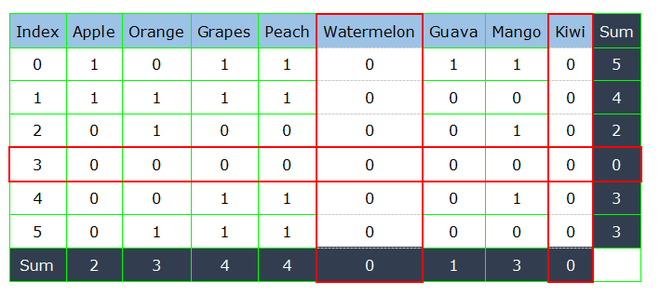## Python3

 `# importing pandas library``import` `pandas as pd`` ` `# creating dataframe``df ``=` `pd.DataFrame({``'Apple'``: [``1``, ``1``, ``0``, ``0``, ``0``, ``0``],``                   ``'Orange'``: [``0``, ``1``, ``1``, ``0``, ``0``, ``1``],``                   ``'Grapes'``: [``1``, ``1``, ``0``, ``0``, ``1``, ``1``],``                   ``'Peach'``: [``1``, ``1``, ``0``, ``0``, ``1``, ``1``],``                   ``'Watermelon'``: [``0``, ``0``, ``0``, ``0``, ``0``, ``0``],``                   ``'Guava'``: [``1``, ``0``, ``0``, ``0``, ``0``, ``0``],``                   ``'Mango'``: [``1``, ``0``, ``1``, ``0``, ``1``, ``0``],``                   ``'Kiwi'``: [``0``, ``0``, ``0``, ``0``, ``0``, ``0``]})`` ` `print``(``"Dataframe before filtering\n"``)``print``(df)`` ` `# filtering on the basis of rows``df ``=` `df[df.``sum``(axis``=``1``) > ``0``]`` ` `# filtering on the basis of columns``df ``=` `df.loc[:, df.``sum``(axis``=``0``) > ``0``]`` ` `print``(``"\nDataframe after filtering\n"``)``print``(df)`

Output: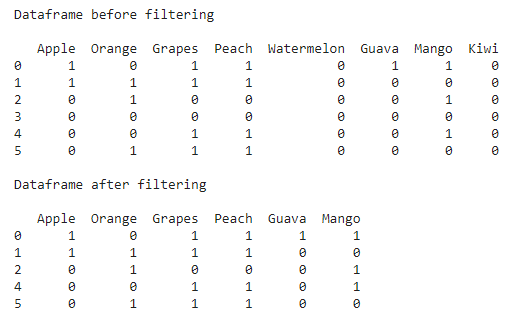### Filtering rows on basis of thesum of few columns

Now if we want to filter those customers who did not buy either of the fruits from a limited list, for example, customers who did not buy either grape, guava, or peach should be removed from the data frame. Here, we filter the rows on the basis of certain columns which are grape, peach, and guava in this case.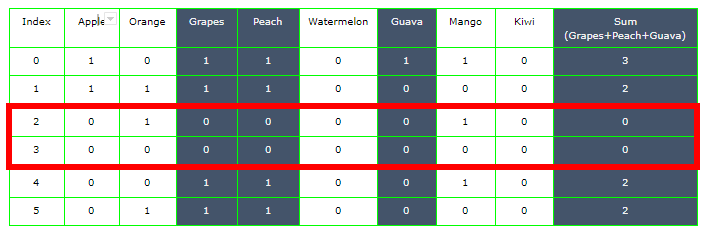On calculating the sum of all rows for these three columns, we find the sum to be zero for indexes 2 and 3.

## Python3

 `# importing pandas library``import` `pandas as pd`` ` `# creating dataframe``df ``=` `pd.DataFrame({``'Apple'``: [``1``, ``1``, ``0``, ``0``, ``0``, ``0``],``                   ``'Orange'``: [``0``, ``1``, ``1``, ``0``, ``0``, ``1``],``                   ``'Grapes'``: [``1``, ``1``, ``0``, ``0``, ``1``, ``1``],``                   ``'Peach'``: [``1``, ``1``, ``0``, ``0``, ``1``, ``1``],``                   ``'Watermelon'``: [``0``, ``0``, ``0``, ``0``, ``0``, ``0``],``                   ``'Guava'``: [``1``, ``0``, ``0``, ``0``, ``0``, ``0``],``                   ``'Mango'``: [``1``, ``0``, ``1``, ``0``, ``1``, ``0``],``                   ``'Kiwi'``: [``0``, ``0``, ``0``, ``0``, ``0``, ``0``]})`` ` `print``(``"Dataframe before filtering\n"``)``print``(df)`` ` `# list of columns to be considered``columns ``=` `[``'Grapes'``, ``'Guava'``, ``'Peach'``]`` ` `# filtering rows on basis of certain columns``df ``=` `df[df[columns].``sum``(axis``=``1``) > ``0``]`` ` `print``(``"\nDataframe after filtering\n"``)``print``(df)`

Output: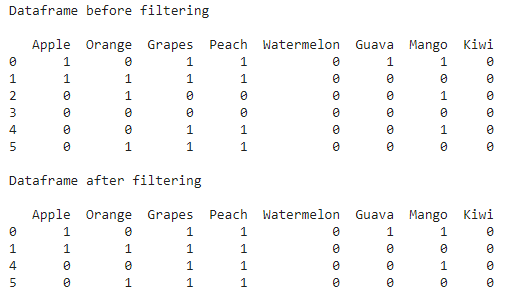Filtering few columns from the entire dataset on the basis of their sum

If you want to remove any of the columns from a list of columns that has sum equals to zero. We only sum those columns and apply the condition on them.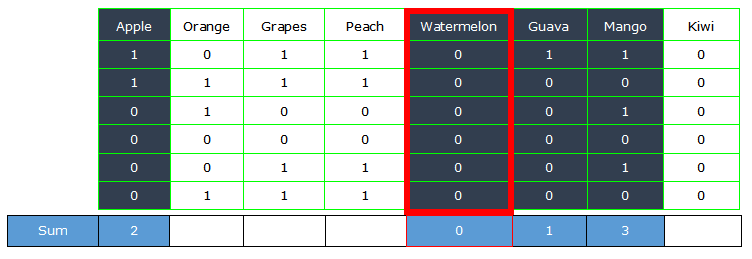## Python3

 `# importing pandas library``import` `pandas as pd`` ` `# creating dataframe``df ``=` `pd.DataFrame({``'Apple'``: [``1``, ``1``, ``0``, ``0``, ``0``, ``0``],``                   ``'Orange'``: [``0``, ``1``, ``1``, ``0``, ``0``, ``1``],``                   ``'Grapes'``: [``1``, ``1``, ``0``, ``0``, ``1``, ``1``],``                   ``'Peach'``: [``1``, ``1``, ``0``, ``0``, ``1``, ``1``],``                   ``'Watermelon'``: [``0``, ``0``, ``0``, ``0``, ``0``, ``0``],``                   ``'Guava'``: [``1``, ``0``, ``0``, ``0``, ``0``, ``0``],``                   ``'Mango'``: [``1``, ``0``, ``1``, ``0``, ``1``, ``0``],``                   ``'Kiwi'``: [``0``, ``0``, ``0``, ``0``, ``0``, ``0``]})`` ` `print``(``"Dataframe before filtering\n"``)``print``(df)`` ` `# list of columns to be considered``columns ``=` `[``'Apple'``, ``'Mango'``, ``'Guava'``, ``'Watermelon'``]`` ` `# iterating through the columns and dropping``# columns with sum less than equals to 0``for` `column ``in` `columns:``    ``if` `(df[column].``sum``() <``=` `0``):``        ``df.drop(column, inplace``=``True``, axis``=``1``)`` ` `print``(``"\nDataframe after filtering\n"``)``print``(df)`

Output: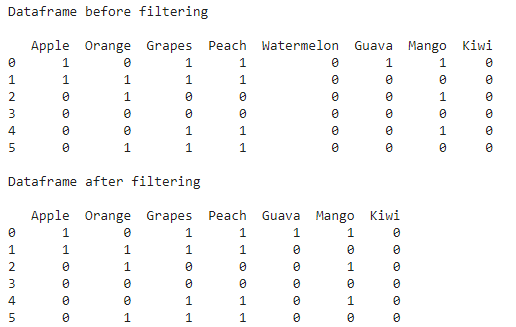In this way, we can modify our data frame in Pandas according to some situations by applying some conditions on rows and columns.

Attention geek! Strengthen your foundations with the Python Programming Foundation Course and learn the basics.

To begin with, your interview preparations Enhance your Data Structures concepts with the Python DS Course. And to begin with your Machine Learning Journey, join the Machine Learning – Basic Level Course

My Personal Notes arrow_drop_up